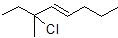# 7.6: The SN1 Reaction

$$\newcommand{\vecs}{\overset { \rightharpoonup} {\mathbf{#1}} }$$ $$\newcommand{\vecd}{\overset{-\!-\!\rightharpoonup}{\vphantom{a}\smash {#1}}}$$$$\newcommand{\id}{\mathrm{id}}$$ $$\newcommand{\Span}{\mathrm{span}}$$ $$\newcommand{\kernel}{\mathrm{null}\,}$$ $$\newcommand{\range}{\mathrm{range}\,}$$ $$\newcommand{\RealPart}{\mathrm{Re}}$$ $$\newcommand{\ImaginaryPart}{\mathrm{Im}}$$ $$\newcommand{\Argument}{\mathrm{Arg}}$$ $$\newcommand{\norm}{\| #1 \|}$$ $$\newcommand{\inner}{\langle #1, #2 \rangle}$$ $$\newcommand{\Span}{\mathrm{span}}$$ $$\newcommand{\id}{\mathrm{id}}$$ $$\newcommand{\Span}{\mathrm{span}}$$ $$\newcommand{\kernel}{\mathrm{null}\,}$$ $$\newcommand{\range}{\mathrm{range}\,}$$ $$\newcommand{\RealPart}{\mathrm{Re}}$$ $$\newcommand{\ImaginaryPart}{\mathrm{Im}}$$ $$\newcommand{\Argument}{\mathrm{Arg}}$$ $$\newcommand{\norm}{\| #1 \|}$$ $$\newcommand{\inner}{\langle #1, #2 \rangle}$$ $$\newcommand{\Span}{\mathrm{span}}$$

##### Objectives

After completing this section, you should be able to

1. write an expression relating reaction rate and reactant concentration for a first-order reaction.
2. compare the kinetics of SN1 and SN2 reactions.
3. identify the rate-limiting step for a reaction, given the reaction energy diagram.
4. sketch a reaction energy diagram for a reaction, given the mechanism and sufficient information to identify the rate-limiting step.
5. write the mechanism of a typical SN1 reaction, and discuss the important features of the mechanism.
6. discuss the stereochemistry of an SN1 reaction, and explain why a racemic mixture is expected when substitution takes place at the chiral carbon atom of an optically pure substrate.
7. explain why unimolecular nucleophilic substitution at the chiral carbon atom of an optically pure substrate does not result in complete racemization.
8. compare the stereochemical consequences of the SN1 mechanism with those of the SN2 mechanism.
##### Key Terms

Make certain that you can define, and use in context, the key terms below.

• first-order reaction
• rate-limiting step
• SN1
##### Study Notes

You should recognize that certain compounds (notably tertiary alkyl halides) which react very slowly by the SN2 mechanism can undergo rapid nucleophilic substitution by an alternative mechanism.

The abbreviation SN1 refers to “unimolecular nucleophilic substitution.” In a first-order reaction, the rate of the reaction depends on the concentration of only one of the reactants. Thus, when an alkyl halide reacts by an SN1 mechanism, the rate of reaction is dependent on the concentration of the alkyl halide, but is independent of the concentration of the attacking nucleophile.

Note: In many textbooks the “rate-limiting step” is called the “rate-determining step.”

Racemization problems can be a potential source of confusion. Most students entering an introductory organic chemistry course have a reasonable background in mathematics, and feel comfortable if they have a formula or equation they can use in this type of situation. Thus, we recommend that you consider the following approach.

1. In a given mixture of enantiomers, let x = the fraction of the (+)-enantiomer, and 1 − x = the fraction of the (−)-enantiomer. [Remember that the fraction can be obtained by dividing the percentage by 100%.]
2. The observed [α]D of the mixture is then given by:

Observed [α]D = x ([α]D of the (+)-enantiomer)

+ (1 − x)([α]D of the (−)-enantiomer)

Another source of confusion is the way in which the terms “percentage racemization” and “percentage inversion” are used. You must be clear in your mind that 80% racemized means that we have 40% of the original configuration and 60% (40% + 20%) of the inverted configuration. This point is illustrated in the diagram below.## General SN1 Reaction

When looking at the following substitution reaction it would be expected to be extremely slow. The reaction represents a worst case scenario based off the rules of an SN2 reaction. The substrate is sterically hindered, the nucleophile is relatively weak, and the solvent is polar protic. However, the reaction proceeds quickly and prefers tertiary substrates over methyl, which is opposite to the trend seen in SN2 reactions. It makes sense that this substitution reaction occurs using a different mechanism than SN2.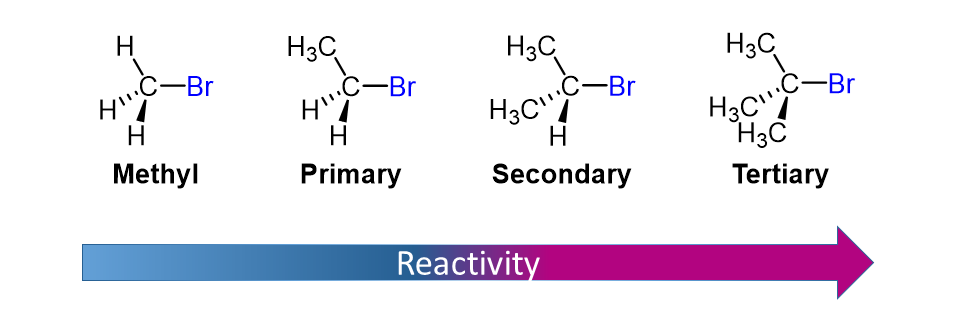### The SN1 mechanism

A second model for a nucleophilic substitution reaction is called the 'dissociative', or 'SN1' mechanism. In the SN1 reaction, the bond between the substrate and the leaving group is broken when the leaving group departs with the pair of electrons that formerly composed the bond.This results in the formation of a carbocation (from "carbon" and "cation") the word for a positively charged carbon atom.  Because the carbocation has only three bonds, it bears a formal charge of +1. Recall that a carbocation is sp2 hybridized, with trigonal planar geometry. The positive charge is contained in an empty, unhybridized p orbital perpendicular to the plane formed by the three sigma bonds. The formation of a carbocation is not energetically favored, so this step in the reaction is the slowest step and determines the overall rate of the reaction. The step which controls the overall rate of a reaction is called the rate-determining step.In the second step of this two-step reaction, the nucleophile attacks the empty, 'electron hungry' p orbital of the carbocation to form a new bond and return the carbon to tetrahedral geometry. Since the nucleophile attacks the carbocation only after the leaving group has departed, there is no need for back-side attack. The carbocation and its substituents are all in the same plane, meaning that the nucleophile can attack from either side. As a result, both enantiomers are formed in an the SN1 reaction, leading to a racemic mixture of both enantiomers.Because SN1 reactions almost exclusively involve neutral nucleophile the product of this second step of the mechanism is often positively charged. The neutral substitution product is usually formed after a third, deprotonation step.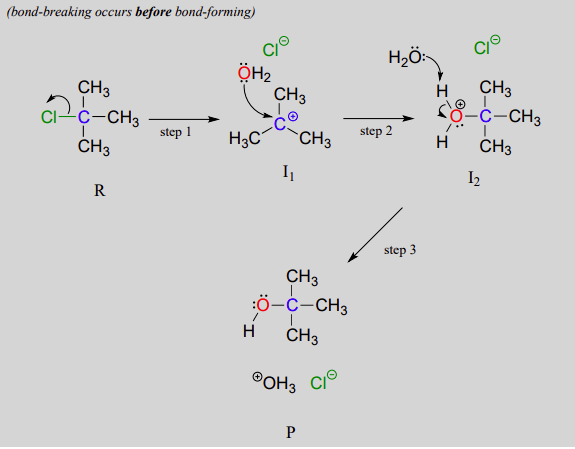### Reaction Coordinate Diagrams and SN1 Mechanism

During the SN1 reaction we see an example of a reaction intermediate, a very important concept in the study of organic reaction mechanisms that was introduced earlier in the module on organic reactivity Recall that many important organic reactions do not occur in a single step; rather, they are the sum of two or more discreet bond-forming / bond-breaking steps, and involve transient intermediate species that go on to react very quickly. In the SN1 reaction, the carbocation species is a reaction intermediate. A potential energy diagram for an SN1 reaction shows that the carbocation intermediate can be visualized as a kind of valley in the path of the reaction, higher in energy than both the reactant and product but lower in energy than the two transition states. The following reaction coordinate diagram shows an example of a an SN1 reaction with a negatively charged nucleophile.There are several important consequences to the unimolecular nature of the rate-determining step in the the SN1 reaction. The first step (dissociation) is the rate determining step, so the rate is controlled only by the loss of the leaving group and does not involve any participation of the nucleophile. Therefore, the rate of the reaction is dependent only on the concentration of the substrate, not on the concentration of the nucleophile.

This can also be shown for the reactions with a neutral nucleophile which includes an extra step of deprotonation. A potential energy diagram for this mechanism shows that each of the two positively-charged intermediates (I1 and I2) can be visualized as a valley in the path of the reaction, higher in energy than both the reactant and product but lower in energy than the transition states.  The first, bond-breaking step is the slowest, rate-determining step - notice it has the highest activation energy and leads to the highest-energy species, I1, the carbocation intermediate. Step 2 is rapid: a new covalent bond forms between a carbocation and a water nucleophile, and no covalent bonds are broken. The third step represents a Bronsted-Lowry proton transfer which is rapid, with low activation energy.Because the first step of the mechanism is the rate determining step, the first transition state will be the most important.  During the first step, a carbon-leaving group bond in a neutral substrate is broken to form a carbocation intermediate and a leaving group anion. The breaking bond is represented as a dashed-partial bond in the transition state. A partial positive charge is shown on the carbon to represent the formation of a carbocation and a partial negative charge is shown on the leaving group to represent the formation of an anion.## SN1 Reaction Kinetics

In the first step of an SN1 mechanism, two charged species are formed from a neutral molecule. This step is much the slower of the three steps, and is therefore rate-determining. In the reaction energy diagram, the activation energy for the first step is higher than that for the second step indicating that the SN1 reaction has first order, unimolecular kinetics because the rate determining step involves one molecule splitting apart, not two molecules colliding. It is important to remember that first order refers to the rate law expression where the generic term substrate is used to describe the alkyl halide.

rate = k [substrate]

Because an SN1 reaction is first order overall the concentration of the nucleophile does not affect the rate.  Since the nucleophile is not involved in the rate-limiting first step, the nature of the nucleophile does not affect the rate of an SN1 reaction.

## Stereochemical Considerations

We saw that reactions result in inversion of stereochemical configuration at the carbon center. The difference in mechanisms means the stereochemical outcome of can be different than SN2. Recall that a carbocation intermediate produced during an SN1 reaction is -hybridized, with an empty p orbital perpendicular to the plane formed by the three sigma bonds: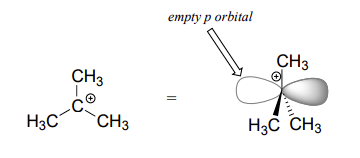Because the nucleophile is free to attack from either side of the carbocation electrophile, the reaction leads to a 50:50 mixture of two stereoisomeric products. In other words: the SN1 reaction occurs with both retention or inversion of configuration at the electrophilic carbon, leading to racemization if the carbon is chiral after the substitution.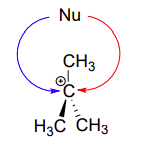However, often chemical reactions are more complicated than the mechanisms that represent limiting cases like SN1 and SN2. Experimental data has shown that the inversion product is favored over racemization due to the formation of ion pairs. After dissociation during the rate determining step the carbocation and the leaving group are still electrostatically associated. The leaving group anion is temporarily held in place which provides a shield to one side of the carbocation. If substitution occurs before the leaving group anion fully diffuses away the stereochemical inversion substitution product is created. Only after the leaving group fully diffuses away can racemization occur. Overall, the product of an SN1 reaction shows an excess of the stereochemical inversion product.For an example, consider the hydrolysis of (S)-3-chloro-3-methylhexane.Exercise $$\PageIndex{1}$$

1)  Consider two nucleophilic substitutions that occur uncatalyzed in solution. Assume that reaction A is SN2, and reaction B is SN1. Predict, in each case, what would happen to the rate of the reaction if the concentration of the nucleophile were doubled, while all other conditions remained constant.2)  Give the products of the following SN1 reaction. Show stereochemistry.3)  Predict with compound in each pair will undergo an SN1 reaction more quickly.

a)or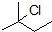b)or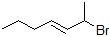c)ord)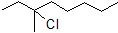or

1) For Reaction A, the rate law is rate = k[CH3I][CH3S-]. Therefore, if the concentration of the nucleophile, CH3S-, is doubled and the concentration of the alkyl halide remains the same, then the reaction rate will double.

For Reaction B, the rate law is rate = k[CH3)3Br]. Therefore, if the concentration of the nucleophile, CH3SH, is doubled and the concentration of the alkyl halide remains the same, then reaction rate stays the same.

2)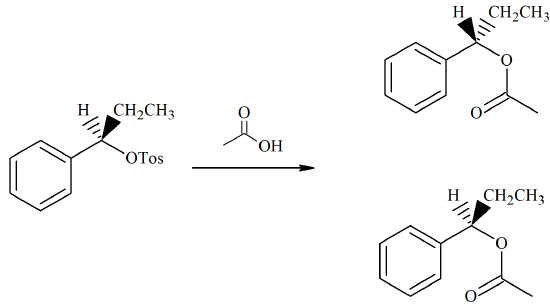3)

a)b)c)d)